Brauer homomorphism

The Brauer homomorphism was first used by R. Brauer [a1], [a2], and developed further by H. Nagao [a4] to establish a connection between the-blocks of a finite groupand of the-local subgroups (cf. Block). Subsequently, it has been used in a variety of other contexts within modular representation theory (cf. also Finite group, representation of a).

Letbe a field of characteristic, and letact by conjugation on the group algebra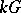with fixed points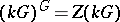. The primitive idempotents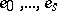incorrespond to the blocks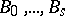of, so that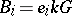andas a direct sum of indecomposable two-sided ideals.

If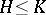are subgroups ofandis a-module, there is a relative trace mapping, given by, where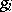runs through a set of coset representatives of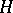in. One writes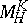for the image of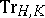. Applying this to the conjugation action ofon, one says thatis a defect group for the block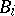(cf. also Defect group of a block) if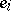lies in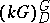but not in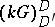for any proper subgroup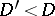. The defect groups of a block form a single conjugacy class of-subgroups of.

Ifis any-subgroup of, still acting onby conjugation, then there is a decomposition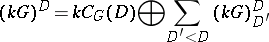as a direct sum of a subring and a two-sided ideal. This is because the left-hand side has as a basis the orbit sums. The orbits of length one span, and the sums of orbits of length greater than one are transfers from proper subgroups of. The Brauer homomorphism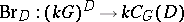is the projection onto the first factor in the above decomposition. Restricting to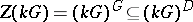, one obtains the homomorphism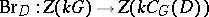originally considered by Brauer.

Brauer's first main theorem states that the Brauer homomorphismestablishes a one-to-one correspondence between the blocks ofwith defect groupand the blocks of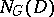with defect group. To make sense of this, first observe that every idempotent in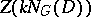lies in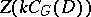(because the other summand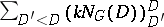is contained in the Jacobson radical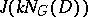). A block idempotent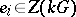has defect groupif and only if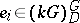and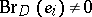. In the commutative diagram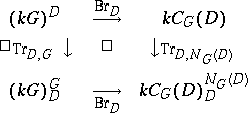the top- and right-hand mappings are surjective, and hence so is the bottom mapping. It therefore induces the bijection of block idempotents described in the first main theorem.

In subsequent work, the Brauer homomorphism has been generalized to arbitrary modules: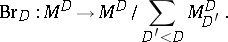The concept is especially useful ifis a-algebra. L. Puig [a5], [a6] has developed an extensive theory of "interior G-algebras" , whereis acting via inner automorphisms, and in which the Brauer homomorphism plays a central role. His work has had extensive impact both on module theory and on block theory, especially his classification of nilpotent blocks [a7]. Further discussion of the Brauer homomorphism in this context can be found in [a3] and [a8].